Get instant live expert help with Excel or Google Sheets“My Excelchat expert helped me in less than 20 minutes, saving me what would have been 5 hours of work!”

#### Post your problem and you'll get expert help in seconds

Your message must be at least 40 characters
Our professional experts are available now. Your privacy is guaranteed.

# Pad a number with zeros

While working with Excel, we are able to pad a number with zeros by using the TEXT and REPT functions, or by simply changing the format of the number.  This step by step tutorial will assist all levels of Excel users in padding a number with zeros in three different methods.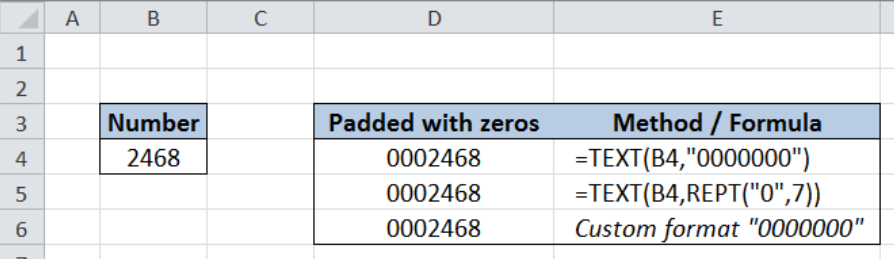Figure 1. Final result:  Pad a number with zeros

Method 1 formula: `=TEXT(B4,"0000000") `

Method 2 formula: `=TEXT(B4,REPT("0",7)) `

Method 3: Using the Custom format “0000000”

## Syntax of the TEXT Function

TEXT converts a number into text

`=TEXT(value, format_text)`

• value – any numeric value, a formula or cell reference to a numeric value
• format_text –  a numeric format expressed as a text string and enclosed in quotation marks

## Syntax of the REPT Function

REPT repeats text for a specified number of times

`=REPT(text, number_times)`

• text – the text we want to repeat
• number_times –  the number of times to repeat the text; must be a positive number

## Setting up our Data

Our data is the number “2468” in cell B4.  We want to pad this number with zeros such that the resulting value has seven digits.  We will record the padded number in cell D4.

In this example, we will learn to pad a number with zeros by using three different methods:

• Using TEXT function
• Using TEXT and REPT functions
• Customizing the format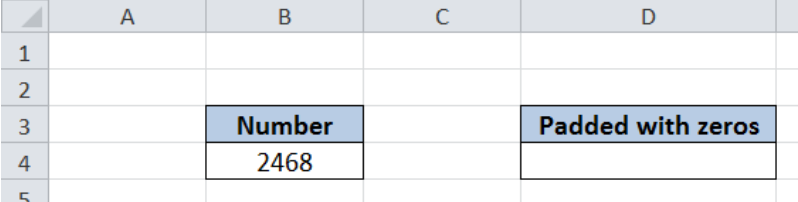Figure 2. Sample data to pad a number with zeros

## Pad a number with zeros using TEXT function

Padding a number with zeros using the TEXT function can be done in three easy steps:

Step 1.  Select cell D4

Step 2.  Enter the formula: `=TEXT(B4,"0000000")`

Step 3.  Press ENTERFigure 3. Entering the formula using TEXT function

Our formula converts the number in cell B4 into a text with the format “0000000”, which means 7 digits.  In this case, the number we want to convert has fewer digits than the zeros in the format.  Hence, TEXT displays insignificant zeros to be able to return seven digits as per our given format.

As a result, the padded number in cell D4 is “0002468”.

## Pad a number with zeros using TEXT and REPT function

There is an alternative solution to padding a number with zeros by combining the TEXT and REPT functions in one formula.  Let us follow these steps:

Step 1.  Select cell D4

Step 2.  Enter the formula: `=TEXT(B4,REPT("0",7))  `

Step 3.  Press ENTER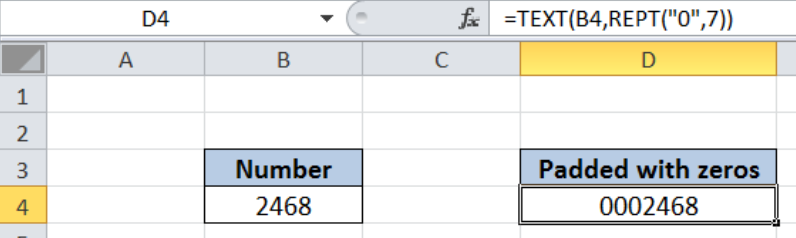Figure 4. Entering the formula using TEXT and REPT functions

Our formula converts the number in cell B4 into a text with the given format.  Our format s determined by the REPT function: REPT(“0”,7).  The REPT formula is another way to represent the format we want.  In this case, REPT returns a text string with the digit zero “0” repeated seven times “0000000”, which is the same as in the previous example.

As a result, the padded number in cell D4 is “0002468”.

The REPT function is especially useful when the number of digits we want for our padded number is variable.  We can link the argument number_times  with a cell reference or a formula.

## Pad a number with zeros by custom format

The first two methods we have learned both convert the number into a text value.  When we want our padded number to remain as a numeric value, we can simply customize the format by following these steps:

Step 1.  Select cell D4

Step 2.  Enter our value “2468”.

Step 3.  Press Ctrl + 1 to launch the Format Cells dialog box.

Step 4.  In the Format Cells preview pane, select Custom.

Step 5.  In the Type box, enter the format “0000000

Step 6.  Press Enter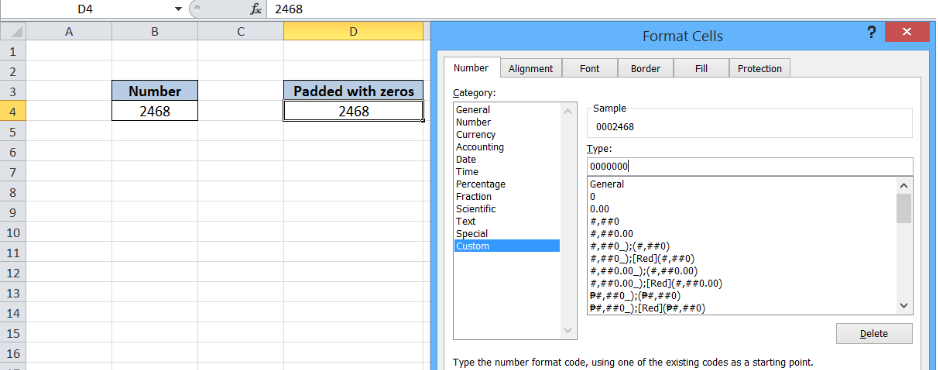Figure 5. Customizing the format to pad a number with zeros

The resulting number still has the value “2468” but it is displayed as “0002468”, which is the same as in the previous methods.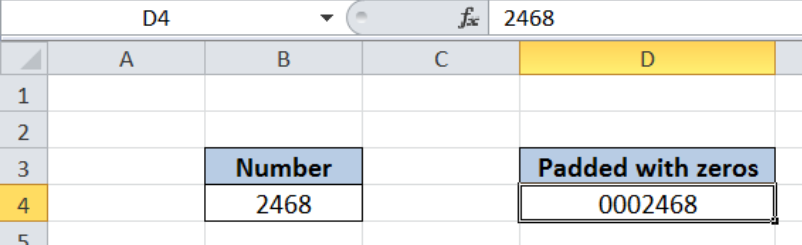Figure 6. Output: Pad a number with zeros by using custom format

The table below shows the three methods having the same final result.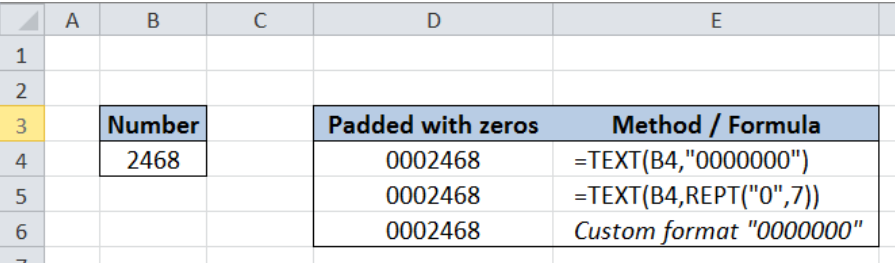Figure 7. Three different methods to pad a number with zeros

Most of the time, the problem you will need to solve will be more complex than a simple application of a formula or function. If you want to save hours of research and frustration, try our live Excelchat service! Our Excel Experts are available 24/7 to answer any Excel question you may have. We guarantee a connection within 30 seconds and a customized solution within 20 minutes.

### Did this post not answer your question? Get a solution from connecting with the expert.Another blog reader asked this question today on Excelchat:
Related blogs## Subscribe to Excelchat.coAnother blog reader asked this question today on Excelchat: